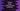# Python break, continue and pass statement : Python Tutorial 15## Break and continue :

In our last tutorial, we have seen that we can use “else” with “for” and “while” loop. Using “break”, we can stop the execution of a code block inside a loop. The control will next move to the next line after the body of the loop. If we use “break” inside a inner loop, the control will move to the outer loop.Let’s take a look into the python break , continue and pass statements :

For the below example,

``````for x in range(100):
print "x = ",x
if x == 2 :
break;
print "for loop ended.."``````

The output will be :

``````x =  0
x =  1
x =  2
for loop ended..``````

i.e. the “for” loop will run for x = 0,1 and 2. and on x=2, it will exit and print the next line after “for” loop.

Now, check for the following example :

``````for x in range(100):
print "x = ",x
for y in range(2):
print "y = ",y
if y == 2 :
break;
break;
print "for loop ended.."``````

The output will be :

``````x =  0
y =  0
y =  1
for loop ended..``````

At first x is 0 for the outer “for” loop.Next it will move to the inner “for” loop. Inner “for” loop will run for y = 0 and y = 1. On y = 2, it will exit the inner “for” loop and finally it will also exit from the outer “for” loop.

“break” also works in the same way for “while” loop :

``````x = 0

while(True):
x = x+1

print "x = ",x

if x == 2:
break;``````

output :

``````x =  1
x =  2``````

## Continue statement :

On using “continue” statement, the control will move to the next iteration of the loop.

``````for x in range(0,10):
if x % 2 == 0 :
print "even no = ",x
continue

print "odd no = ",x``````

output :

``````even no =  0
odd no =  1
even no =  2
odd no =  3
even no =  4
odd no =  5
even no =  6
odd no =  7
even no =  8
odd no =  9``````

If the number is even, the control will move to the next “for” loop iteration. So it will not print the next line .

## Pass statement :

“pass” statement does nothing. The only difference between a comment and a pass statement is that the interpreter ignores the comment but it will not ignore the pass statement. Suppose , you want to create a for loop , but you don’t want to implement, you can leave a pass statement with it.

``````for x in range(0,10):
pass``````

The above code will not run.